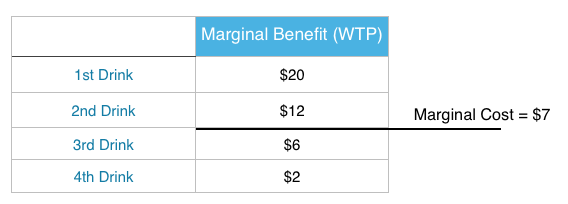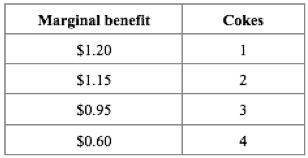Topic 1: Introductory Concepts and Models

# 1.3 Marginal Analysis

### Learning Objectives

By the end of this section, you will be able to:

• Use marginal analysis to determine the right quantity of an action
• Calculate marginal net benefit of an additional unit of activity

“Economics is the painful elaboration of the obvious” – Anonymous.

That quote might seem quite relevant when the biggest conclusion of our last section was that you should do something if the benefits outweigh the costs. While sometimes economics can seem obvious, it is important to first understand how a rational consumer should behave before seeing how we fail to meet that standard.

## Marginal Analysis

In the last section we showed how to make a binary decision, but not all decisions fit that category. Many are ‘how much’ decisions. For example, if you have decided to go clubbing, how many drinks do you buy? This is a decision where we use marginal analysis. Marginal analysis is the process of breaking down a decision into a series of ‘yes or no’ decisions. More formally, it is an examination of the additional benefits of an activity compared to the additional costs incurred by that same activity.

To make a decision using marginal analysis, we need to know the willingness to pay for each level of the activity. As mentioned, this is also known as the marginal benefit from an action.

To decide how many drinks to buy, you have to make a series of yes or no decisions on whether to buy an additional drink. In Table 1.3a the marginal benefit is diminishing. This means that you are willing to pay more for the 1st drink than the next. Your friends are all drinking, so you are likely willing to pay quite a lot for your 1st drink. By the 4th, you may feel as though you do not need another.

So how many drinks will you buy if the cost is \$7? To make this decision, we must use marginal analysis for each level. This means comparing our marginal benefit with marginal cost of an additional unit of activity. In this case marginal cost is just equal to \$7.For the 1st Drink: MB = \$20 > MC = \$ 7, you should buy the drink.

For the 2nd Drink: MB = \$12 > MC = \$ 7, you should buy the drink.

For the 3rd Drink: MB = \$6 < MC = \$ 7, you should not buy the drink.

With this simple process we can easily see that you will buy 2 drinks, unless there is a price change.

## Net Benefit

What is our net benefit from the actions, or how much ‘happiness’ have we gained? To calculate, all we have to do is add up our benefits and subtract our costs.

Total Benefit = \$20 + \$12 = \$32

Total Cost = \$7 + \$7 = \$14

Net Benefit = \$32 – \$14 = \$18

It is important to recognize that our act of marginal analysis has maximized this benefit. Consider what would happen if we purchased 3 drinks.

Total Benefit = \$20 + \$12 + \$6 = \$38

Total Cost = \$7 + \$7 + \$7 = \$21

Net Benefit = \$38 – \$21 = \$17

Note that although total benefit is more than it was previously, net benefit is lower. Looking closer we can see that net benefit fell because our total costs rose (\$14 –> \$21) by more than our total benefits (\$32 –> \$38). As a quick rule:

When total benefits rise more than total costs, then the action is logical.

When total costs rise more than total benefits, then the action is illogical.

This is why we look at the marginal net benefit of a decision, rather that the total. It is as though all the previous actions are ‘sunk’. We can calculate the marginal net benefit of a decision by subtracting marginal cost from marginal benefit. Marginal net benefit of the first drink is \$13 (\$20 – \$7), the 2nd is \$5 (\$12 – \$7), and the third is -\$1 (\$6 – \$7). As long as the marginal net benefit is positive, we should increase our activity!

## Summary

Marginal analysis is an essential concept for everything we learn in economics, because it lies at the core of why we make decisions. We have just scratched the surface of it now, but will go more in depth in Topic 3. For now, we will turn our attention to a slightly different topic – trade.

## Glossary

Marginal Analysis The examination of the additional benefits of an activity compared to the additional costs incurred by that same activity

Marginal Benefit

The additional satisfaction one gains from an additional unit of an activity

Marginal Cost

Marginal Net Benefit
The difference between the marginal benefits and marginal costs of an action

### Exercises 1.3

1. According to marginal analysis, optimal decision-making involves:

a) Taking actions whenever the marginal benefit is positive.
b) Taking actions only if the marginal cost is zero.
c) Taking actions whenever the marginal benefit exceeds the marginal cost.
d) All of the above.

2. Jane’s marginal benefit per day from drinking coke is given in the table below. This shows that she values the first coke she drinks at \$1.20, the second at \$1.15, and so on.
If the price of coke is \$1.00, the optimal number of cokes that Jane should drink is:a) 1.
b) 2.
c) 3.
d) 4.